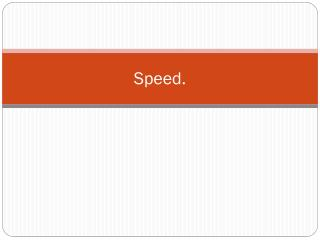DownloadDownload PresentationSpeed.

# Speed.

Download Presentation## Speed.

- - - - - - - - - - - - - - - - - - - - - - - - - - - E N D - - - - - - - - - - - - - - - - - - - - - - - - - - -
##### Presentation Transcript

1. Speed.

2. What is speed? • Speed is the distance travelled per unit time. • Speed has the units of m/s or km/h.

3. Formula • The formula for speed is v=d/t. • The formula can be arranged in a triangle to help rearrange the equation. d v t

4. Example • Jonny travels to the store 30m from his house, it takes him 40seconds to walk there. • What speed did Jonny walk at? • v=d/t • v=30/40 • v=0.75m/s

5. Questions. • A fielder throws a ball to the wicket keeper, the fielder was 40meters from the keeper when he threw the ball it takes the ball 4seconds to reach the keeper. • What is the speed the ball travels?

6. Questions • A car travels for 5hours at 75km/h. • How far does the car travel in this time? • It takes Sophie 300seconds to walk to the shop 150meters from her house. • At what speed does Sophie walk?

7. Questions • Shae travels on his bike for an hour at 35km/h he then reach a hill that takes him half an hour to bike up at 15km/h. • How far does Shae travel in the hour and a half?

8. Questions. • Convert 60km/h into m/s? • Convert 28m/s into km/h? • If you run at 12m/s. How long would it take you to run 100m?

9. Questions.